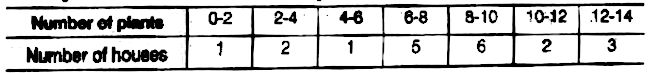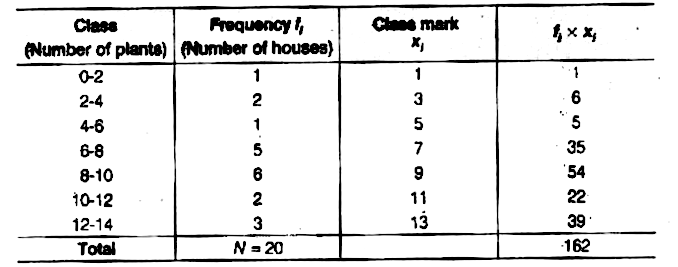Home/Class 10 Math Chapter List/6. Statistics/

# A survey was conducted by a group of students as a part of their environment awareness programme, in which they collected the following data regarding the number of plants in 20 houses in a locality, Find the mean number of plants per house.Which method did you use for finding the mean and why?

## QuestionMathClass 10

A survey was conducted by a group of students as a part of their environment awareness programme, in which they collected the following data regarding the number of plants in 20 houses in a locality, Find the mean number of plants per house.Which method did you use for finding the mean and why?

Let us find the mean of the data by direct method because the values involved in the given data are small.We have, $$N=\sum\limits _{i=1}^{7}f_{i}$$=20 and $$\sum\limits _{i=1}^{7}f_{i}x_{i}=162$$
Then, mean of the data is
$$\overline {x}=\frac {1}{N}\times \sum\limits _{i=1}^{7}f_{i}x_{i}$$
$$=\frac {1}{20}\times 162=8.1$$
Hence, the required mean of the data is$$8.1$$plants.Here, we have used direct method because numerical values of $$x_{i}$$and$$f_{i}$$are small.